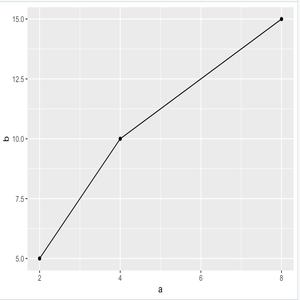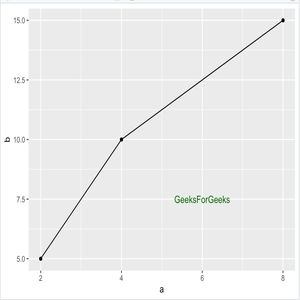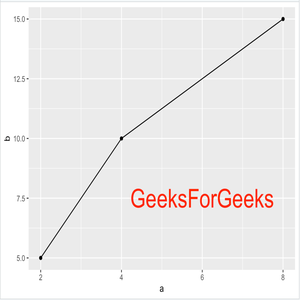Related Articles
Change Font Size for Annotation using ggplot2 in R
• Last Updated : 03 Mar, 2021

ggplot2 is a data visualization package for the statistical programming language R. After analyzing and plotting graphs, we can add an annotation in our graph by annotate() function. This article discusses how the font size of an annotation can be changed with the annotation() function.

Syntax: annotate()

Parameters:

• geom : specify text
• x : x axis location
• y : y axis location
• label : custom textual content
• color : color of textual content
• size : size of text
• fontface : fontface of text
• angle : angle of text

By adding annotate function with only argument geom=’text’, it shows that ggplot knows that it has to add text, but it needs another parameter such as Location of text (x,y) and text data (label=text).

Approach

• Import ggplot2
• Create/Import Dataset
• Plot the data on a graph
• Add annotation() function with required parameters

Let us first create a basic plot without annotating.

Program :

## R

 `# Import Package``library``(ggplot2)`` ` `# df dataset``df <- ``data.frame``(a=``c``(2,4,8),             ``                 ``b=``c``(5, 10, 15))`` ` `# plot graph``plot = ``ggplot``(df, ``aes``(x = a, y = b)) + ``geom_point``() + ``geom_line``()`` ` `# output``plot`

Output:Basic Plot

Now, Let us see how annotation can be added.

Program :

## R

 `# Import Package``library``(ggplot2)`` ` `# df dataset``df <- ``data.frame``(a=``c``(2,4,8),             ``                 ``b=``c``(5, 10, 15))`` ` `# plot graph``plot = ``ggplot``(df, ``aes``(x = a, y = b)) + ``geom_point``() + ``geom_line``()`` ` `plot + ``annotate``(``'text'``, ``                ``x = 6, y = 7.5, ``                ``label = ``'GeeksForGeeks'``,``                ``color=``'darkgreen'``)`

Output:label & color

To change the size of the text, use the “size” argument. In the below example, the size of GeeksForGeeks is 10 and the color is red.

Program :

## R

 `# Import Package``library``(ggplot2)`` ` `# df dataset``df <- ``data.frame``(a=``c``(2,4,8),             ``                 ``b=``c``(5, 10, 15))`` ` `# plot graph``plot = ``ggplot``(df, ``aes``(x = a, y = b)) + ``geom_point``() + ``geom_line``()`` ` `plot + ``annotate``(``'text'``, x = 6, y = 7.5, ``                  ``label = ``'GeeksForGeeks'``,``                  ``color=``'red'``,``                  ``size = 10)`

Output:Size

Attention reader! Don’t stop learning now. Get hold of all the important DSA concepts with the DSA Self Paced Course at a student-friendly price and become industry ready.

My Personal Notes arrow_drop_up